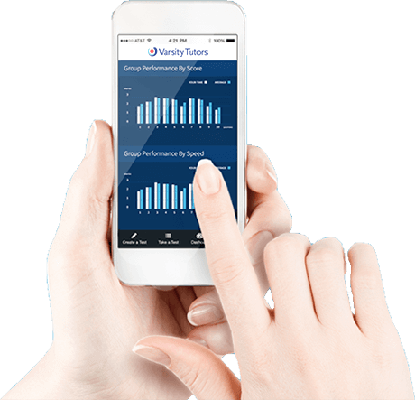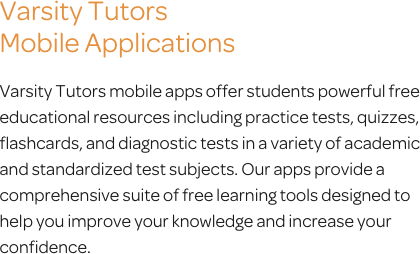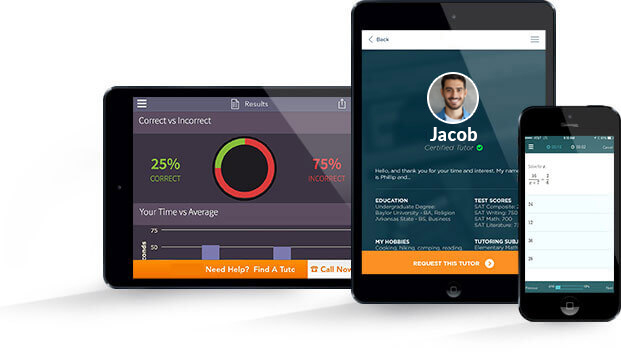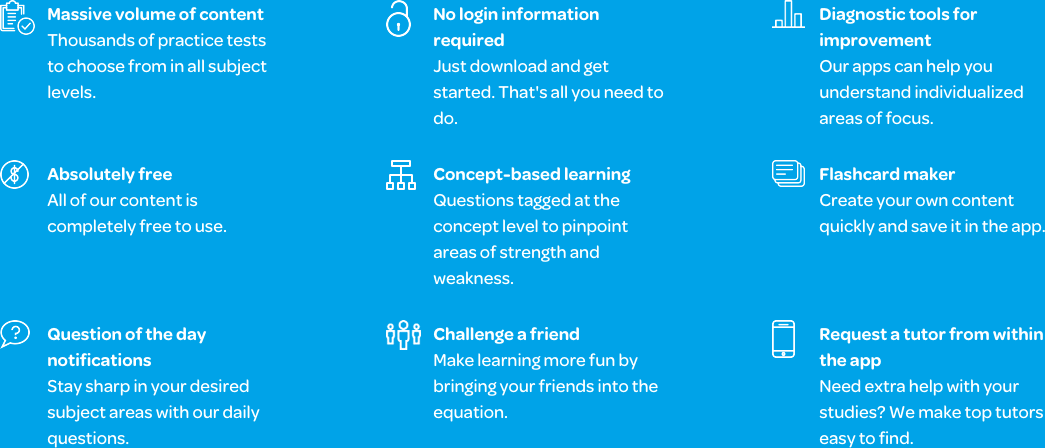# Varsity Tutors Common Core Math 3rd Grade mobile app### The Varsity Tutors Common Core Math 3rd Grade Mobile App

If your child is a student in a 3rd-grade mathematics class or is taking a Common Core 3rd Grade Math exam, they might need some supplemental study material to gain the proficiency needed to succeed. The Varsity Tutors Common Core 3rd Grade Math mobile learning app, available free of charge on iTunes and the Google Play Store, is a great way to help them get the assistance needed to understand difficult concepts covered in their math class. While the topics covered in the Common Core curriculum for 3rd-grade mathematics can vary from state to state, this app works to cover the most frequently covered material, thus ensuring your child understands all of the important concepts in the course. This means that they will learn multiplication and division, fractions, finding the area of rectangles, and begin to touch on various geometric subjects.

One of the best features of the Common Core 3rd Grade Math mobile learning app is that you can use it any time you want. As long as you have an iPad, iPhone, or Android device nearby, you can easily access the material covered in the subject. This means that helping your child study math is easy and convenient. Furthermore, the program has a variety of different tools that serve a wide range of functions. For example, the Question of the Day feature is great for parents who want to give their child a quick, one-question study session. This feature presents a new problem every day and is an excellent way to help a pupil keep 3rd-grade mathematics in the forefront of their mind.

For more comprehensive study plans, there are the flashcards and Learn by Concept features. Both cover the bulk of the curriculum but act in different ways. Learn by Concept is treated as an interactive syllabus, where subjects are broken down into different categories that can be reviewed like an interactive textbook. Flashcards, on the other hand, are a great way to drill information and are also arranged by topics.

When you feel that your child has received sufficient revision, simply have them take one of the practice tests available on the mobile app. There are a number of topic-specific practice tests that evaluate their knowledge of categories of 3rd-grade math, like geometry, measurements, or numbers and operations. These practice tests are short in length and can be completed in a few minutes. Full-Length Practice tests, on the other hand, are comprehensive and are designed to emulate an actual test. They will evaluate your child’s understanding of the subject as a whole, and they are much longer than their counterparts. Both tests come with an answer key that helps to explain how each right answer was found, the time it took to complete the question, and how they performed compared to others who took the test.

By making use of the Varsity Tutors Common Core 3rd Grade Mathematics mobile learning app, you are working to give your child the assistance needed to succeed. Unlike textbooks, which are difficult to transport, this study program is easy to bring with you. By doing so, you are able to use any moment to begin a study session.Further, students are expected to understand fractions, rectangular arrays, two-dimensional shapes, and areas. Each of these skills builds the foundation for future courses. There are many connections that third-grade students are expected to make during their coursework, each of which links the concepts they learn with one another. You can explore these connections further by downloading the Varsity Tutors Common Core Grade 3 app from iTunes or the Google Play Store.

Each concept introduced in third-grade math is required understanding to do well on the final exam. The class will work with division and multiplication, as well as build upon their understanding of subtraction and addition. They will begin working with more complex problems. The students will learn to manipulate arithmetic using multiple numbers, as well as develop an understanding of fractions and their interaction with numbers. Furthermore, third-graders begin using geometric measurements and equations while working with time, liquid, and mass. It is additionally important that students learn to think rationally while approaching shapes. By the end of the course, each student must be able to demonstrate a deep understanding of these concepts and their applications. By the end of the course, third-graders should not only be able to solve the problems they are facing, but understand the reasoning and logic behind them.

The final exam at the end of the year expects third-grade students be able to display their grasp of the information they learned. The Common Core Grade 3 Math exam assesses their ability to solve problems using mathematical concepts, while also understanding the reasoning behind them. In the exam, students will face two-part word problems that call for the use of the four mathematical equations. They will need to understand fractions and the way they correlate to number lines, as well as to tell time. They will need to be able to take apart shapes and use them to solve problems, as well as repeatedly demonstrate reasoning. You can prepare your child for their final exam with Varsity Tutors’ free Common Core Grade 3 Math app, available for iPads, iPhones, and Android devices.

Common Core Grade 3 Math focuses on problem-solving. Students are expected to develop an understanding of division and multiplication. They will be tasked with interpreting various equations into different contexts, such as visually. Students will need to be capable of working with whole and partial numbers up to 100, as well as to solve word problems with their newfound division and multiplication skills. They will be required to demonstrate their reasoning by explaining it with imagery, equations, or symbols. Further, they’ll have to be able to use these equations to solve for an unknown variable to solve problems.

Third-grade students will need to have a firm grasp of the properties and relationships between division and multiplication. It is important that they know how to apply various strategies that allow one to quickly solve problems. The most important strategies that students learn in third-grade math are the associative, commutative, and distributive properties. These are the properties of operations. Depending on if they are multiplying or dividing, they will need to use different ones. In addition, they will need to be able to use multiplication to solve division as an unknown-factor equation, which means they can solve a division problem by identifying the digit that makes the appropriate number when multiplied by the other number.

Multiplication and division share a relationship that allows students to apply the properties of operations to their equations. Third-grade students are expected to comprehend these properties, as well as how they can be implemented with division and multiplication in word and equation problems. Simply put, every math system a student will work with follows the rules of one of these properties. The distributive property allows each child to be able to solve problems that use factoring. They can easily understand that “ab + at” is interchangeable with “a(b + t).” The associative property allows students to regroup equations to make them easier to approach. The rule is that “a + (b + c) = (a + b) + c.” The commutative property moves digits around without changing the formula, like “a + b = b + a.” It is additionally imperative that the student understands the correct situations in which to use each property.

In third grade, students begin learning to solve multiplication and division problems that use numbers up to 100. There are numerous strategies that each child can implement to learn to be fluent in the arithmetic. They must be able to understand how to solve a multiplication problem by understanding the divisional relationship between the digits the student is given.

All four basic math operations are important for Common Core Grade 3 Math. Students will need to be able to solve word problems using subtraction, addition, division, and multiplication. They must be able to use letters for unknown variables, demonstrate reasoning with mental math, use estimation strategies fluently, and perform other strategies for two-step problems. Furthermore, they must be able to explain the order of operations while identifying important mathematical patterns with ease. Each student should be able to quickly figure out the right strategies to implement for the most accurate results, while understanding the reasoning behind the choice. The free Common Core Grade 3 Math app from Varsity Tutors covers gives you plenty of practice with these concepts, and you can download it right now from iTunes and the Google Play Store.

Common Core Grade 3 Math expects a student to gain a thorough mastery of algebraic equations and relationships. In order for a child to succeed in the future, these are valuable strategies, skills, and formulas to grasp. Before moving on to fourth-grade math, students should achieve a high mastery of the concepts at hand. At the end of the year, they will be given a final exam that is designed to assess their development. In addition, many schools provide regular assessment testing to determine each student’s mastery over the coursework along the way. Supplement your child’s preparation for this testing by downloading Varsity Tutors’ Common Core Grade 3 Math app today for your iPhone, iPad, or Android device.